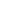# Free CBSE Class 4 Maths Division Worksheets

Download free printable Division Worksheets to practice. With thousands of questions available, you can generate as many Division Worksheets as you want.

## Sample CBSE Class 4 Maths Division Worksheet Questions

1.

Match the following:

 a).  6 X 7 = 42, 42/6 = 7, 42/7 = 6 1).  Divisor b).  35/5 = 7, 5 x 7 = 35, 7 X 5 = 35 2).  250 c).  500/2 3).  Multiplication Fact d).  Dividend is greater than 4).  Division fact e).  Remainder is smaller than 5).  Quotient

2.
How many hours are there in 300 minutes?
3.
The cost of 7 pens is Rs 84. Find the cost of each pen?
4.
The cost of 5 hairbands are Rs.50. Find the cost of each hairband?
5.
The product of two numbers 880. If one number is 10. Find the other number?
6.
A train covers a distance 820 km in 8 hours. How much distance is covered in 1 hour?
7.
924 apples were packed in 35 boxes. How many apples were packed in each box?
8.
642 bags of sugar equally loaded in 6 trucks. How many bags were loaded in each truck?
9.
How many hours are there in 420 minutes?
10.
870 balls are equally packed in 3 boxes. How many balls are packed in 1 box?

## Find more Division Worksheets

Worksheets by UrbanPro

Our worksheets are designed to help students explore various topics, practice skills and enrich their subject knowledge, to improve their academic performance. Designed by Experts who have extensive experience and expertise in teaching a subject, these worksheets will improve your child's problem-solving skills and subject knowledge in a fun and interactive manner.
Check out our free customized worksheets across school boards, grades, subjects and levels of subject knowledge. You can download, print and share these worksheets with anyone, anywhere, anytime!

Get a custom worksheet to practice!

Select your topic & see the magic.

subjectSelect Chapter(s)

Chapters & Subtopics